# Highlights

The authors find two properties of neural networks.

• There is no distinction between individual high-level units and random linear combinations of high-level units.
• Existence and transferability of adversarial examples.

# On the units

Let $$x \in \mathbb{R}^m$$ be an input image and $$\phi(x)$$ the activation values of some layer. One can look at what inputs maximize the features of $$\phi(x)$$, that is: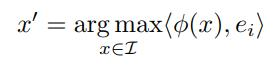The authors find that many images that satisfy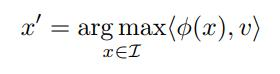are semantically related to each other, where $$v$$ is a random vector.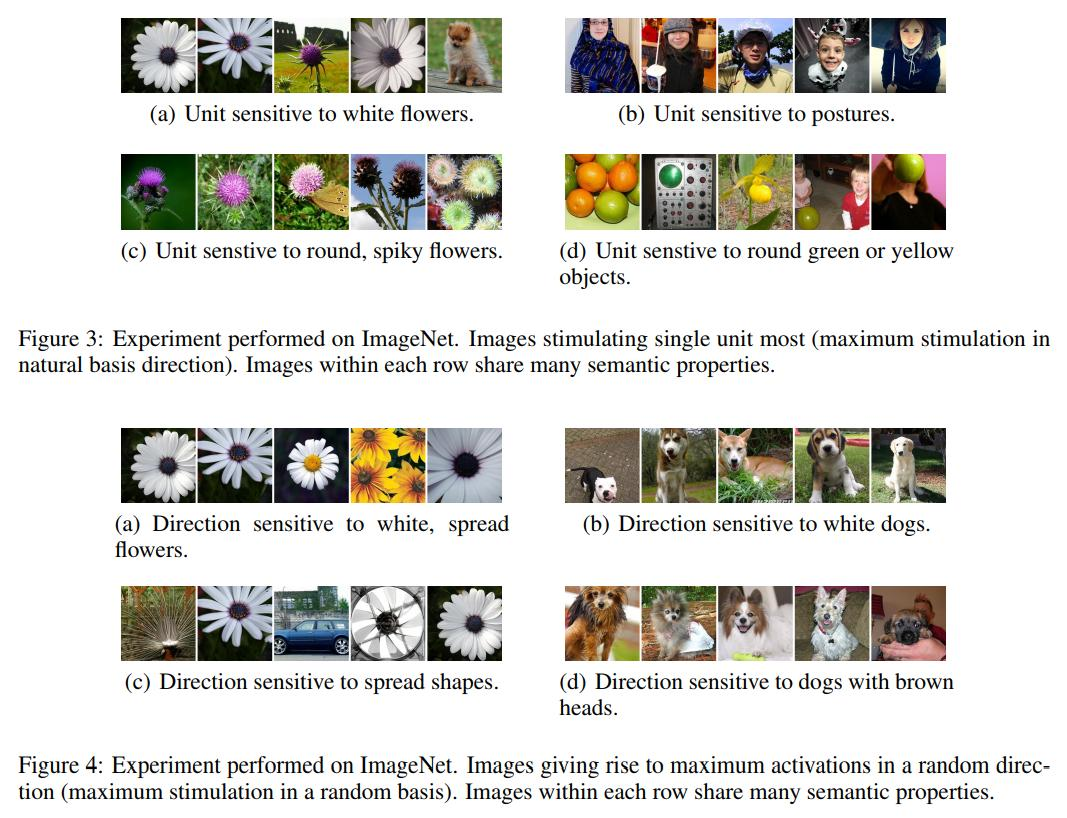• This puts into question the notion that neural networks disentangle variation factors across coordinates.

Let $$f:\mathbb{R}^m \to \{1,...,k \}$$ be a classifier with an associated loss function. For a given input $$x \in \mathbb{R}^m$$ and target label $$l \in \{1,...,k \}$$, the aim is to solve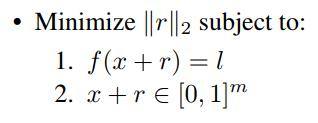The minimizer is denoted $$D(x,l)$$. This task is non-trivial only if $$f(x) \not= l$$. The authors find an approximation of $$D(x,l)$$ by line-search to find the minimum $$c>0$$ for which the minimum $$r$$ of the following problems atisfies $$f(x+r)=l$$.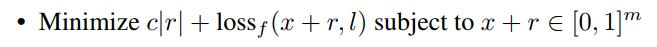• In the convex case this yields the exact solution.

# Experiments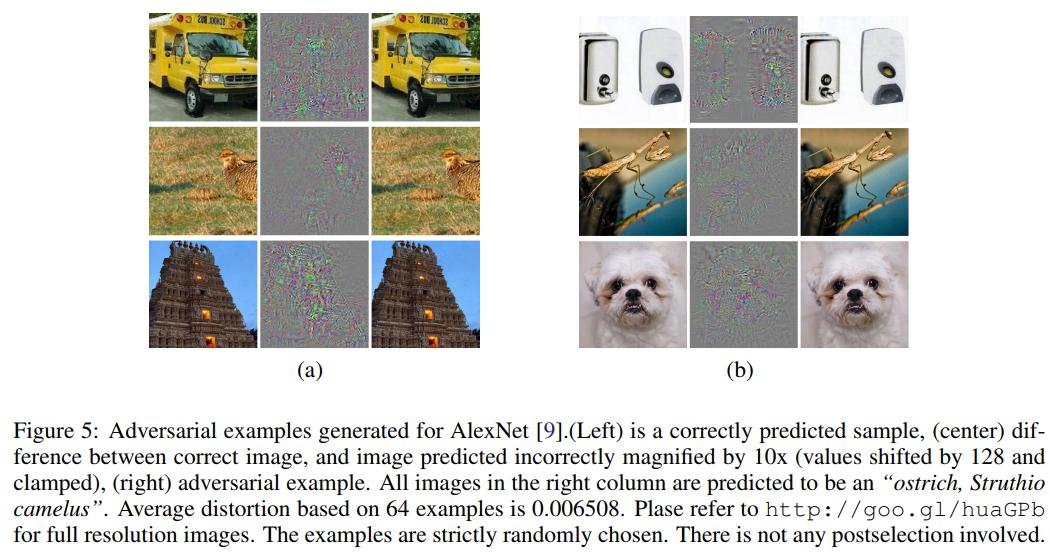• Adversarial examples transfer to other architectures trained from scratch with different hyperparameters: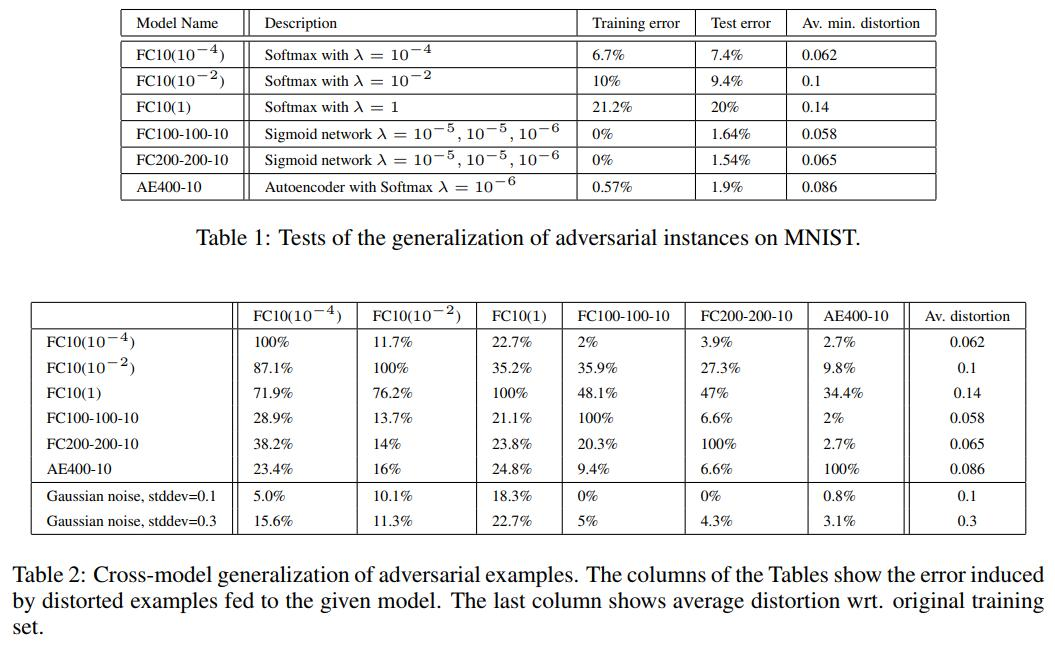• Adversarial examples transfer to other architectures trained on a disjoint training set: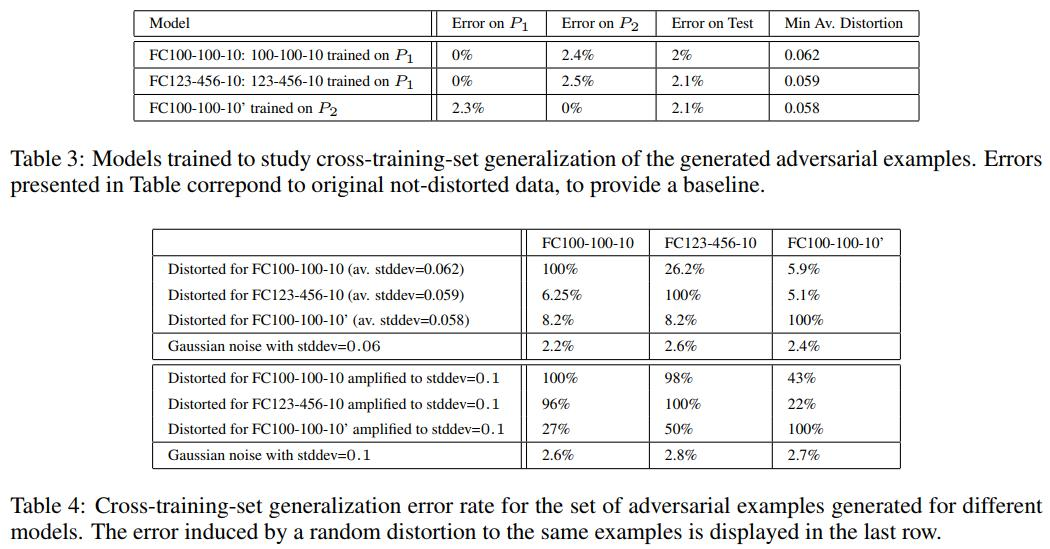• Adding random noise to the input images is not as effective to misclassify samples when compared to adversarial examples generation: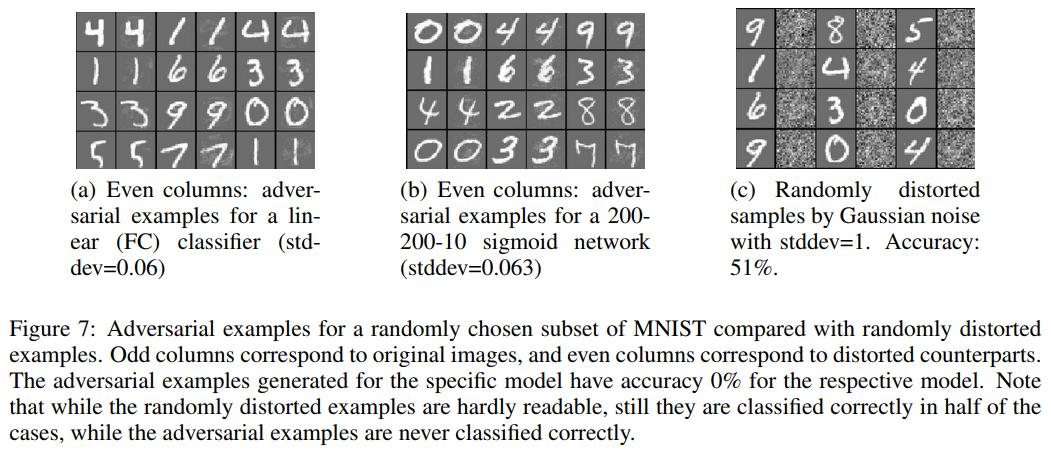# Theoretical analysis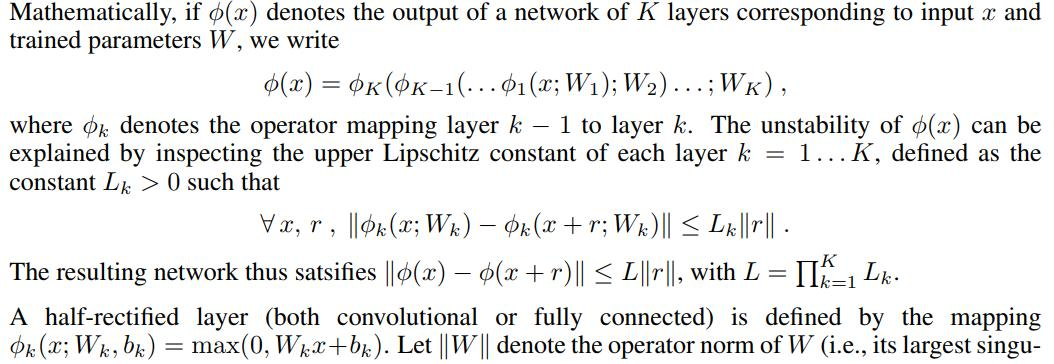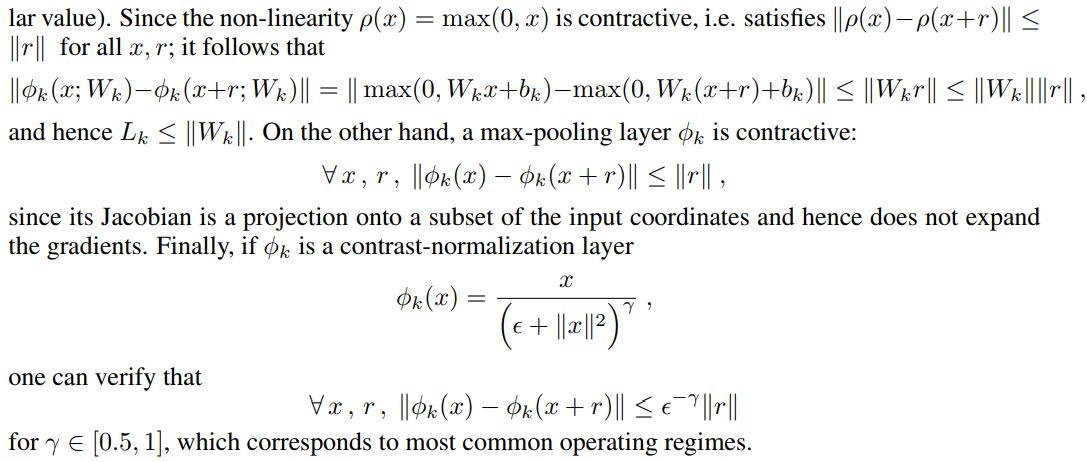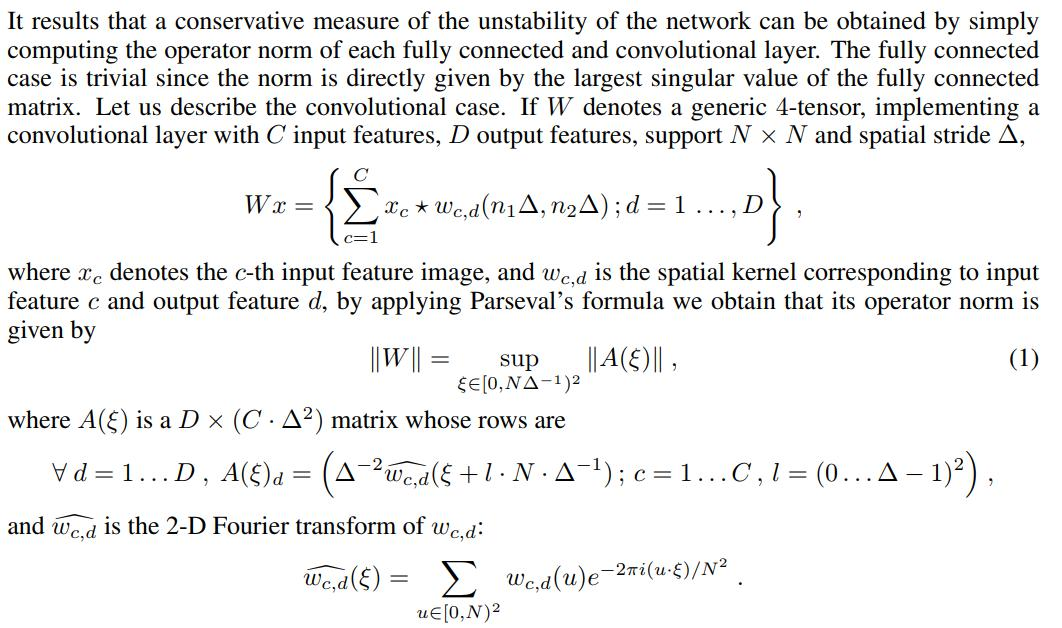# Conclusion

• The semantic meaning of activations of neurons is not meaningful since random directions in feature space present similar properties.
• Adversarial examples can be found for any neural network, they transfer across architectures trained with different hyperparameters and even different data sets.